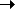Package dom.core Class public class TypeInfo Inheritance TypeInfoObject

 Introduced in: DOM 3 Core

The TypeInfo interface represents a type referenced from Element or Attr nodes, specified in the schemas associated with the document. The type is a pair of a namespace URI and name properties, and depends on the document's schema.

Note: This is not implemented in Mozilla.

Public Properties
PropertyDefined By
DOM 3 Core typeName : DOMString
[read-only] The name of a type declared for the associated element or attribute, or null if unknown.
TypeInfo
DOM 3 Core typeNamespace : DOMString
[read-only] The namespace of the type declared for the associated element or attribute or null if the element does not have declaration or if no namespace information is available.
TypeInfo
Public Methods
MethodDefined By

DOM 3 Core isDerivedFrom(typeNamespaceArg:DOMString, typeNameArg:DOMString, derivationMethod:Number):Boolean
This method returns if there is a derivation between the reference type definition, i.e.
TypeInfo
Public Constants
ConstantDefined By
DOM 3 Core DERIVATION_EXTENSION : Number = 2
[static] If the document's schema is an XML Schema, this constant represents the list.
TypeInfo
DOM 3 Core DERIVATION_LIST : Number = 8
[static] If the document's schema is an XML Schema [XML Schema Part 1], this constant represents the union if simple types are involved.
TypeInfo
DOM 3 Core DERIVATION_RESTRICTION : Number = 1
[static] If the document's schema is an XML Schema, this constant represents the derivation by extension.
TypeInfo
DOM 3 Core DERIVATION_UNION : Number = 4
[static] If the document's schema is an XML Schema, this constant represents the derivation by restriction if complex types are involved, or a restriction if simple types are involved.
TypeInfo
Property Detail
 DOM 3 Core typeName property
`typeName:DOMString`  [read-only]

 Introduced in: DOM 3 Core

The name of a type declared for the associated element or attribute, or null if unknown.

Implementation
`    public function get typeName():DOMString`

 DOM 3 Core typeNamespace property
`typeNamespace:DOMString`  [read-only]

 Introduced in: DOM 3 Core

The namespace of the type declared for the associated element or attribute or null if the element does not have declaration or if no namespace information is available.

Implementation
`    public function get typeNamespace():DOMString`

Method Detail
 DOM 3 Core isDerivedFrom () method
` public function isDerivedFrom(typeNamespaceArg:DOMString, typeNameArg:DOMString, derivationMethod:Number):Boolean`

 Introduced in: DOM 3 Core

This method returns if there is a derivation between the reference type definition, i.e. the TypeInfo on which the method is being called, and the other type definition, i.e. the one passed as parameters.

Parameters

 `typeNamespaceArg:DOMString` — The namespace of the other type definition. `typeNameArg:DOMString` — The name of the other type definition. `derivationMethod:Number` — The type of derivation and conditions applied between two types, as described in the list of constants provided in this interface.

Returns
 `Boolean` — If the document's schema is a DTD or no schema is associated with the document, this method will always return false. If the document's schema is an XML Schema, the method will true if the reference type definition is derived from the other type definition according to the derivation parameter. If the value of the parameter is 0 (no bit is set to 1 for the derivationMethod parameter), the method will return true if the other type definition can be reached by recursing any combination of {base type definition}, {item type definition}, or {member type definitions} from the reference type definition.

Constant Detail
 DOM 3 Core DERIVATION_EXTENSION Constant
`public static const DERIVATION_EXTENSION:Number = 2`

 Introduced in: DOM 3 Core

If the document's schema is an XML Schema, this constant represents the list.

The reference type definition is derived by extension from the other type definition if the other type definition can be reached recursively following the {base type definition} property from the reference type definition, and at least one of the derivation methods involved is an extension.

 DOM 3 Core DERIVATION_LIST Constant
`public static const DERIVATION_LIST:Number = 8`

 Introduced in: DOM 3 Core

If the document's schema is an XML Schema [XML Schema Part 1], this constant represents the union if simple types are involved.

The reference type definition is derived by list from the other type definition if there exists two type definitions T1 and T2 such as the reference type definition is derived from T1 by DERIVATION_RESTRICTION or DERIVATION_EXTENSION, T2 is derived from the other type definition by DERIVATION_RESTRICTION, T1 has {variety} list, and T2 is the {item type definition}. Note that T1 could be the same as the reference type definition, and T2 could be the same as the other type definition.

 DOM 3 Core DERIVATION_RESTRICTION Constant
`public static const DERIVATION_RESTRICTION:Number = 1`

 Introduced in: DOM 3 Core

If the document's schema is an XML Schema, this constant represents the derivation by extension.

The reference type definition is derived by restriction from the other type definition if the other type definition is the same as the reference type definition, or if the other type definition can be reached recursively following the {base type definition} property from the reference type definition, and all the derivation methods involved are restriction.

 DOM 3 Core DERIVATION_UNION Constant
`public static const DERIVATION_UNION:Number = 4`

 Introduced in: DOM 3 Core

If the document's schema is an XML Schema, this constant represents the derivation by restriction if complex types are involved, or a restriction if simple types are involved.

The reference type definition is derived by union from the other type definition if there exists two type definitions T1 and T2 such as the reference type definition is derived from T1 by DERIVATION_RESTRICTION or DERIVATION_EXTENSION, T2 is derived from the other type definition by DERIVATION_RESTRICTION, T1 has {variety} union, and one of the {member type definitions} is T2. Note that T1 could be the same as the reference type definition, and T2 could be the same as the other type definition.